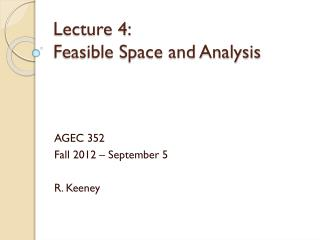DownloadDownload PresentationLecture 4: Feasible Space and Analysis

# Lecture 4: Feasible Space and Analysis

Télécharger la présentation## Lecture 4: Feasible Space and Analysis

- - - - - - - - - - - - - - - - - - - - - - - - - - - E N D - - - - - - - - - - - - - - - - - - - - - - - - - - -
##### Presentation Transcript

1. Lecture 4: Feasible Space and Analysis AGEC 352 Fall 2012 – September 5 R. Keeney

2. Linear Equations & Systems • Recall the following for y = mx + b • Linear equations have constant slope • Differentiate y = mx + b and the result is b • If restrict y and x to be non-negative we are only dealing with the 1st quadrant of the Cartesian plane

3. Linear Equations & Systems • The solution to two linear equations is an (x,y) pair that defines the intersection • Two linear equations also • May have no solution • Identical slope, different intercepts • May have no non-negative solution • Different slope and intercept, intersect outside the 1st quadrant • May have many solutions • Same slope and intercept

4. Production Possibilities Frontier • A producer has a given amount of inputs • Must choose the best quantity of different outputs • Assumptions • Costs are sunk on inputs • Profit will be maximized where revenue is maximized

5. Graph of the PPF Q2 1) How do we interpret the PPF? 2) What does feasible mean in terms of the PPF? 3) How do we solve the economic problem (Revenue maximization) that goes with the PPF? Q1

6. What does the PPF have to do with linear equation systems? • The shape of the PPF is not known in general • Intro economics draws it smooth and bowed out • Because we need to teach 2 things • 1) Elasticity of supply • Requires a smooth curve with a derivative • 2) Declining marginal transformation • Requires that additional units of 1st output given up produce smaller yields of the 2nd output

7. Linear functions may be a good approximation to a PPF • No elasticities but the problem is easier to solve • Can still represent bowed out PPF to a degree Q2 Q1

8. Constraints and inequalities • The PPF is a constraint representing • 1) available technology (ways to turn inputs into outputs) • 2) available quantities of inputs (Z) • It is more appropriately represented as a boundary of the entire feasible set it defines • Why might this be?

9. An example with two outputs • A manufacturer makes two brands of beverages • PF = Premium Finest • SS = Standard Stuff • The manufacturer has three resources available for making the beverages • C = corn (600 bushels) • S = sugar (600 pounds) • M = machinery (200 hours)

10. Technical information • How do the inputs become output? • Some analysis of this information • Identify the most limiting resource for each beverage. • How would we do that?

11. Most limiting resource • The goal is to see what resource is “most scarce” for each product • The “most scarce” resource will drive the economics of the product • Step 1: For each input, divide the total available quantity by the requirement per gallon of the beverage • Step 2: Identify the most limiting as the lowest number (i.e. it limits the beverage quantity to X)

12. Most Limiting cont.

13. Most Limiting cont.

14. Most limiting summary • We will never make more than 120 gallons of PF • We will never make more than 100 gallons of SS • If we make 120 gallons of PF, we make no SS • If we make 100 gallons of SS, we make no PF

15. Feasible Space • Is this the right feasible space?

16. Feasible Space • Identifying the most limiting resource for each output tells us… • 1) the correct endpoints (intersections with the axes) for the feasible space but nothing about the points in between • 2) which inputs are most likely to determine the economics of the optimal output mix • To visualize the feasible space, we need to graph a set of inequalities

17. A Constraint • Corn available is 600 bushels • PF uses 5 bushels per gallon • SS uses 3 bushels per gallon • Need to write a total corn usage inequality • 5*PF + 3*SS ≤ 600 • To graph this we need to • 1) convert it to an equality/equation • 2) identify two points for the equation

18. Corn constraint cont. • 5*PF + 3*SS = 600 • The easiest two points to get from this equation are • 1) when PF = 0 • 2) when SS = 0 • Plug zero in for one of the outputs, solve for the other that solve the equation • We already did that in the most limiting factors so we have • (PF, SS) = {(0,200), (120,0)}

19. Corn constraint cont. • All combinations of PF and SS that can be produced considering only the corn limit • Remember the inequality, everything inside of the line can be produced as well

20. Other constraints • Returning to the most limiting factor analysis we can find two points for each of the other resources as well • Sugar: (PF, SS) = {(0,300), (150,0)} • Machinery: (PF, SS) = {(0,100), (200,0)} • Graph those in the same space as the corn constraint…

21. Feasible Space Questions Which side of the line is feasible and which is infeasible? Which constraints ‘define’ the feasible space? How would we go from feasible analysis to ‘best’ analysis? What combo uses all corn and machinery time?